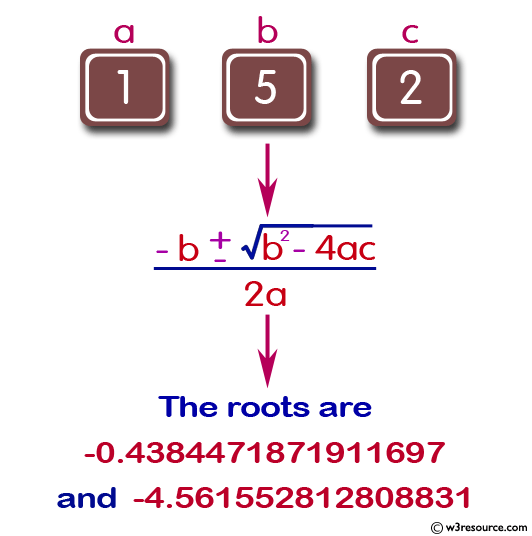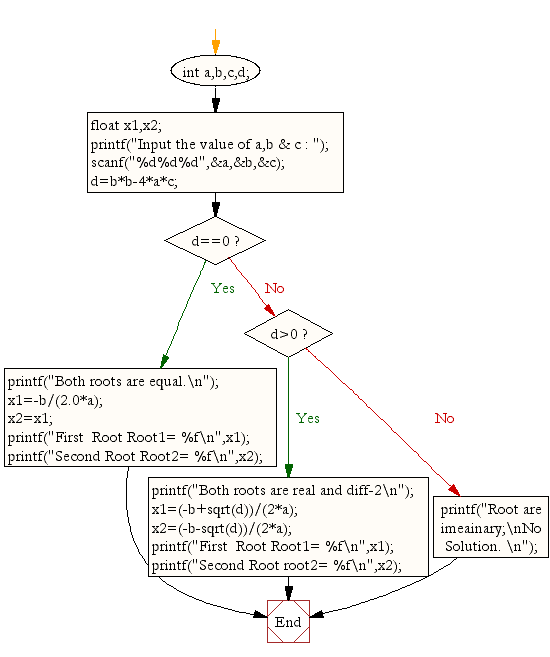﻿ C Program: Calculate the root of a Quadratic Equation - w3resource# C Exercises: Calculate the root of a Quadratic Equation

## C Conditional Statement: Exercise-11 with Solution

Write a C program to calculate the root of a Quadratic Equation.

Pictorial Presentation:Sample Solution:

C Code:

``````#include <stdio.h>
#include <math.h>

void main()
{
int a,b,c,d;
float x1,x2;

printf("Input the value of a,b & c : ");
scanf("%d%d%d",&a,&b,&c);
d=b*b-4*a*c;
if(d==0)
{
printf("Both roots are equal.\n");
x1=-b/(2.0*a);
x2=x1;
printf("First  Root Root1= %f\n",x1);
printf("Second Root Root2= %f\n",x2);
}
else if(d>0)
{
printf("Both roots are real and diff-2\n");
x1=(-b+sqrt(d))/(2*a);
x2=(-b-sqrt(d))/(2*a);
printf("First  Root Root1= %f\n",x1);
printf("Second Root root2= %f\n",x2);
}
else
printf("Root are imeainary;\nNo Solution. \n");
}
```
```

Sample Output:

```Input the value of a,b & c : 1 5 7
Root are imaginary;
No Solution.
```

Flowchart:C Programming Code Editor:

Improve this sample solution and post your code through Disqus.

What is the difficulty level of this exercise?

Test your Programming skills with w3resource's quiz.

﻿

## C Programming: Tips of the Day

Why do C and C++ compilers allow array lengths in function signatures when they're never enforced?

It is a quirk of the syntax for passing arrays to functions.

Actually it is not possible to pass an array in C. If you write syntax that looks like it should pass the array, what actually happens is that a pointer to the first element of the array is passed instead.

Since the pointer does not include any length information, the contents of your [] in the function formal parameter list are actually ignored.

Ref : https://bit.ly/3fhlvdH